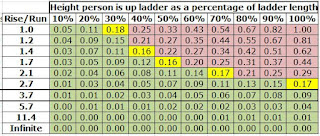## Tuesday, March 21, 2017

In case you were wondering about the origin of the hoary rule-of-thumb that the rise-over-run for a ladder should be 3:1 or 4:1A ladder.  The red arrow is the weight of the person climbing the ladder.  Obviously, that person can be anywhere between the bottom rung and the top of the ladder.  The top of the ladder is treated as a roller.  It can only push perpendicular to the ladder.This is called a free body diagram.  The upper contact point is replaced with a force vector and force vectors are also pencilled in at the bottom.The critical coefficient of friction is the ratio between the vertical force between the ground and the horizontal force that must resist the sliding of the ladder.  In many engineering applications, a coefficient of friction of 0.15 is assumed for smooth, flat surfaces like sheet steel.
Grinding through the math the critical coefficient of friction equals something like Cos(theta)^2*d/l/(1-sin(theta)*cos(theta)*d/l)  where theta is the inside angle of the ladder and the ground.  The math is included in case anybody wants to check my algebra.

Something interesting happens as the person climbs the ladder;  the critical coefficient of friction goes up.  The downward force stays constant but the horizontal force goes up as the person gets closer to the top.2.7:1 is slightly iffy.  3.7:1 is safe.  In case you were wondering about the weird numbers, 2.7:1 is 70% and 3.7:1 is 75 degrees.
The heck of it is that the ladder is perfectly safe...until you get way up high and have much potential energy and then....SURPRISE!

The sweet spot for safety is somewhere in the 3:1 and the 4:1 ratio.  Ratios are used because most guys on the job site do not have a protractor to measure the angle but are very good at eyeballing ratios or distance in feet.